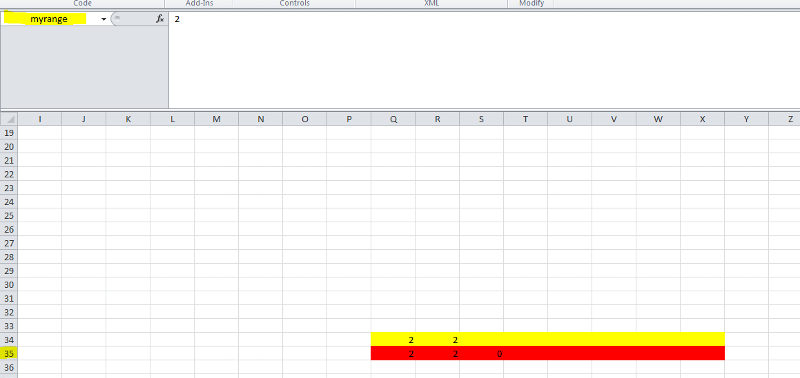What happens with values in a VBA code in Excel 2007 when I insert new rows on the worksheet?hermesalpha used Ask the Experts™
on
I received below code earlier from an EE member here, and now I want to add new rows on this worksheet, but first need to know if that is accounted for automatically in the VBA code? Or would I need to change the VBA to reflect the number of new rows I insert?

The code makes sure that if I first enter a value in the cells referenced to in that code and then delete this value, then the original formula gets restored in the cell.

This is the VBA code:
Option Explicit
Dim KeepValue(1 To 8) As Variant

Private Sub Worksheet_Calculate()
Dim i As Integer
On Error GoTo ErrorOut
Application.EnableEvents = False
For i = 1 To 8
If KeepValue(i) <> Cells(34, i + 15) And Cells(35, i + 15).HasFormula = True Then
Cells(35, i + 15) = Cells(34, i + 15)
End If
KeepValue(i) = Cells(34, i + 15)
Next i
ErrorOut:
Application.EnableEvents = True
End Sub

Private Sub Worksheet_Change(ByVal Target As Range)
If Not Intersect(Target, Range("Q35:X35")) Is Nothing And Target.Count = 1 Then
On Error GoTo ErrorOut
Application.EnableEvents = False
If IsEmpty(Target) Then
Target.FormulaR1C1 = "=IFERROR(R[-1]C,"""")"
End If
ErrorOut:
Application.EnableEvents = True
End If
End Sub
Comment
Watch Question

Do more withEXPERT OFFICE® is a registered trademark of EXPERTS EXCHANGE®
"Batchelor", Developer and EE Topic Advisor
Top Expert 2015
Commented:
The range the VBA code applies to is pretty restricted by "Q35:X35". That range will not change (VBA code does not get adjusted automatically, but Excel formulas used in the sheets are).
The changed cell has to be in exactly that range: Q35 to X35.
Commented:
You can name the range Q35:X35 to something like INPUTRANGE and then refer to it in the code.
Commented:
If you add new row it will not be automatically accounted in your current VBA code, However if you want that to be accounted automatically when a new row is added then you will have to name your range. to Name your range select the range (Q35:X35) then go to the namebox and write myrange as shown in the screenshot below and then press enter.Below is the revised VBA code which automatically account when new row is added.

Option Explicit
Dim KeepValue(1 To 8) As Variant

Private Sub Worksheet_Calculate()
Dim i As Integer
On Error GoTo ErrorOut
Application.EnableEvents = False
For i = 1 To 8
If KeepValue(i) <> Cells(34, i + 15) And Cells(35, i + 15).HasFormula = True Then
Cells(35, i + 15) = Cells(34, i + 15)
End If
KeepValue(i) = Cells(34, i + 15)
Next i
ErrorOut:
Application.EnableEvents = True
End Sub

Private Sub Worksheet_Change(ByVal Target As Range)
If Not Intersect(Target, Range("myrange")) Is Nothing And Target.Count = 1 Then
On Error GoTo ErrorOut
Application.EnableEvents = False
If IsEmpty(Target) Then
Target.FormulaR1C1 = "=IFERROR(R[-1]C,"""")"
End If
ErrorOut:
Application.EnableEvents = True
End If
End Sub

Attached please find an example file.
EE-Name-range.xlsb
Commented:
This is the first code for a specific range from your previous question
http://www.experts-exchange.com/questions/28738819/How-do-I-retrieve-a-value-from-cell-2-to-cell-1-if-cell-2-contains-a-value-and-cell-1-is-empty-but-prioritize-to-let-a-value-be-input-in-cell-2-while-the-formula-in-cell-2-is-protected.html

The question ended with a more flexible code using named ranges.
RestoreRange equals the range here Q35:X35, and CompareRange are the cells above Q34:X34.
It can also work with non contiguous ranges.
If you use that, the code will work when you insert rows above.

Option Explicit
Dim KeepValue() As Variant, KeepFormula() As String
Dim rwMax As Integer, colMax As Integer, j As Integer, k As Integer

Private Sub Worksheet_Calculate()
Dim i As Integer
Dim c As Range
If j <> Range("CompareRange").Count Then
j = Range("CompareRange").Count
ReDim KeepValue(j)
End If
On Error GoTo ErrorOut
Application.EnableEvents = False
i = 0
For Each c In Range("CompareRange")
i = i + 1
If KeepValue(i) <> c.Value And c.Offset(1, 0).HasFormula = True Then
c.Offset(1, 0).Value = c.Value
End If
KeepValue(i) = c.Value
Next c

ErrorOut:
Application.EnableEvents = True
End Sub

Private Sub Worksheet_Change(ByVal Target As Range)
Dim c As Range
Application.EnableEvents = False
On Error GoTo ErrorOut

If Not Intersect(Target, Range("RestoreRange")) Is Nothing And Target.Count = 1 Then
If IsEmpty(Target) Then
Target.FormulaR1C1 = "=IFERROR(R[-1]C,"""")"
End If
End If

If k = 2 Then
If Target.Row > rwMax Or Target.Column > colMax Then
GetFormulas
End If
For Each c In Target
If IsEmpty(c) And KeepFormula(c.Row, c.Column) <> "" Then
c.Formula = KeepFormula(c.Row, c.Column)
ElseIf c.HasFormula = True Then
KeepFormula(c.Row, c.Column) = c.Formula
End If
Next c
Else
GetFormulas
End If
ErrorOut:
Application.EnableEvents = True
End Sub

Private Sub Worksheet_SelectionChange(ByVal Target As Range)
If k = 0 Then
GetFormulas
End If
End Sub

Private Sub GetFormulas()
Dim rw As Integer, col As Integer
rwMax = Cells.SpecialCells(xlCellTypeLastCell).Row
colMax = Cells.SpecialCells(xlCellTypeLastCell).Column
If k = 0 Then
ReDim KeepFormula(1 To rwMax, 1 To colMax)
Else
ReDim Preserve KeepFormula(1 To rwMax, 1 To colMax)
End If
k = 1
On Error GoTo ErrorOut
For rw = 1 To rwMax
For col = 1 To colMax
If Cells(rw, col).HasFormula Then
KeepFormula(rw, col) = Cells(rw, col).Formula
End If
Next col
Next rw
k = 2
ErrorOut:
End Sub
Older than dirt
Most Valuable Expert 2017
Distinguished Expert 2018

Commented:
I've requested that this question be closed as follows:

Accepted answer: 125 points for Excel_Expert's comment #a41385404
Assisted answer: 125 points for ssaqibh's comment #a41384916
Assisted answer: 125 points for Qlemo's comment #a41384913
Assisted answer: 125 points for hgholt's comment #a41385422

for the following reason:

This question has been classified as abandoned and is closed as part of the Cleanup Program. See the recommendation for more details.

Commented:
I suggest to award 150 points to comment 04 and 22 because they included VBA also, and 100 points to comment 16 and 13.

Commented:
I suggest to award 150 points to comment 04 and 22 because they included VBA also, and 100 points to comment 16 and 13.

Do more withSubmit tech questions to Ask the Experts™ at any time to receive solutions, advice, and new ideas from leading industry professionals.